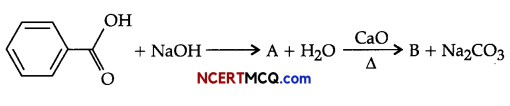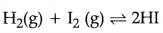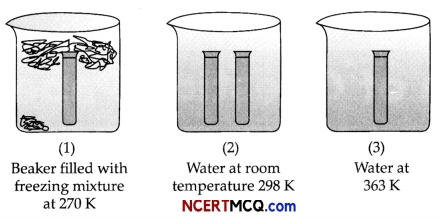Students can access the CBSE Sample Papers for Class 11 Chemistry with Solutions and marking scheme Term 2 Set 5 will help students in understanding the difficulty level of the exam.

## CBSE Sample Papers for Class 11 Chemistry Standard Term 2 Set 5 for Practice

Max. Marks: 40
Time: 2 Hours
General Instructions:

• There are 12 questions in this question paper with internal choice.
• SECTION A-Q. No. 1 to 3 are very short answer questions carrying 2 marks each.
• SECTION B-Q. No. 4 to 11 are short answer questions carrying 3 marks each.
• SECTION C-Q. No. 12 is case based question carrying 5 marks.
• Alt questions are compulsory.
• Use of log tables and calculators is not allowed.

Section – A (2 Marks each)

Question 1.
Arrange the following in the increasing order of their property indicated (Any 2):
(i) Tin, Silicon, Germanium,Carbon (Catenation)
(ii) Aluminium, Gallium, Boron, Thallium, Indium (Ionisation enthalpy)
(iii) Carbon, Tin, Germanium, Silicon (Electronegativity)

Question 2.
What are allotropes? What is the impact of structure on physical properties of two allotropes?

Question 3.
(i) Lithium forms only monoxide where as sodium forms peroxide. Why?
(ii) Second ionisation energy of group 2 elements is less than group 1 elements.

Section – B (3 Marks each)Question 4.
Account for the following:
(i) For an electruphilic substitution reaction, the presence of a halogen atom in the benzene ring affect
reaction.
(ii) An alkene A on ozonolysis gives a mixture of ethanal and pentan-3- one.
(iii)In an electrophiic substitution reaction of nitrobenzene, the presence of nitro group affect the
reaction.
OR
Convert the following:
(i) Benzene into ethylbenzene
(ii) Phenol into Benzene
(iv) Propyne into propane

Question 5.
(i) The pressure of a 1:4 mixture of dihydrogen and dioxygen enclosed in a vessel is one atmosphere. What would be the partial pressure of dioxygen?
(ii) Which are the two wrong assumptions of the kinetic molecular theory of gases which led to the failure of the ideal gas law?
OR
(i) A vessel of 120 ml capacity contains a certain amount at 35°C and 1.2 bar pressure. The gas is transferred to another vessel of volume 180 mL at 35°C. What would be its pressure ?
(ii) Compressibility factor, Z, of a gas is given as Z = $$\frac{\mathrm{PV}}{n \mathrm{RT}}$$
(a) What is the value of Z for an ideal gas ?
(b) For real gas what will be the effect on the value of Z above Boyle’s temperature ?

Question 6.
Account for the following:
(i) Why is the standard enthalpy of formation of diamond not zero although it is an element?
(ii) Why entropy of steam is more than that of water at its boiling point?
(iii) One mole of acetone requires less heat to vaporise than 1 mole of water.Question 7.
An unsaturated hydrocarbon ‘A’ adds two molecules of H2 and on reductive ozonolysis gives butane-1,4- dial, ethanal and propanone. Give the structure of ‘A’, write its IUPAC name and explain the reactions involved.

Question 8.
Observe the figure given below and answer the questions that follow:(i) What does the diagram in the figure used for?
(ii) Derive relationship between ΔH and ΔU.
(iii) Internal energy of a system belongs to which type of property?

Question 9.
What happens when reactions:
(i) m-xylene is treated with Br2 in presence of FeBr3.
(ii) Acetylene is passed through dil. H2SO4 in presence of HgSO4.
(iii) Propene on reaction with Bayer’s reagent.
OR
(i) Write IUPAC names of the following:(ii) Complete the following:Question 10.
An organic base X has K equal to 1 × 10-8. In what amount should 0.01 M HCl and 0.01 M solution of X be mixed to prepare 1 litre of a buffer solution having pH = 7?

Question 11.
(i) For the reaction,the standard free energy is ΔG°> 0. What is the value
of K?
(ii) A solution of Na2CO3 is alkaline. Given reason.
(iii) Ba(OH)2 is soluble in water while BaSO4 is almost insoluble. Given reason.
OR
On the basis of the figure given below, answer the following questions:(i) In which of the figure, forward reaction is favoured?
(ii) What does it tell about the (heat produced/absorbed in the reaction) type of reaction?
(iii) An equilibrium mixture at 300 K contains N2O4 and NO2 at 0.28 and 1.1 atmospheric pressures respectively. If the volume of the container is doubled, calculate the new equilibrium pressure of the two gases.Section – C (5 Marks)

Question 12.